Next: Equilibrium of Floating Bodies Up: Hydrostatics Previous: Hydrostatic Pressure

# Buoyancy

Consider the air/water system described in the previous section. Letbe some volume, bounded by a closed surface, that straddles the plane, and is thus partially occupied by water, and partially by air. The-component of the net force acting on the fluid (i.e., either water or air) contained withinis written (see Section 1.3)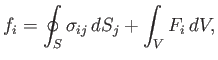(2.5)

where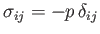(2.6)

is the stress tensor for a static fluid (see Section 1.5), and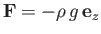(2.7)

the gravitational force density. (Recall that the indices,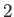, and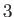refer to the-,-, and-axes, respectively. Thus,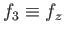, et cetera.) The first term on the right-hand side of Equation (2.5) represents the net surface force acting across, whereas the second term represents the net volume force distributed throughout. Making use of the tensor divergence theorem (see Section B.4), Equations (2.5)-(2.7) yield the following expression for the net force: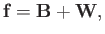(2.8)

where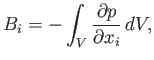(2.9)

and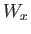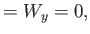(2.10)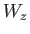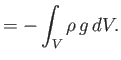(2.11)

Here,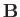is the net surface force, and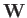the net volume force.

It follows from Equations (2.4) and (2.9) that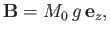(2.12)

where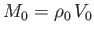. Here,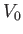is the volume of that part ofwhich lies below the waterline, and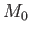the total mass of water contained within. Moreover, from Equations (2.2), (2.10), and (2.11),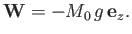(2.13)

It can be seen that the net surface force,, is directed vertically upward, and exactly balances the net volume force,, which is directed vertically downward. Of course,is the weight of the water contained within. On the other hand,, which is generally known as the buoyancy force, is the resultant pressure of the water immediately surrounding. We conclude that, in equilibrium, the net buoyancy force acting acrossexactly balances the weight of the water inside, so that the total force acting on the contents ofis zero, as must be the case for a system in mechanical equilibrium. We can also deduce that the line of action of(which is vertical) passes through the center of gravity of the water inside. Otherwise, a net torque would act on the contents of, which would contradict our assumption that the system is in mechanical equilibrium.Next: Equilibrium of Floating Bodies Up: Hydrostatics Previous: Hydrostatic Pressure
Richard Fitzpatrick 2016-03-31